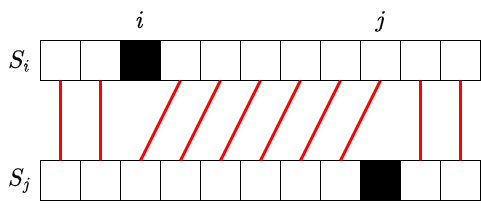Contest Duration: - (local time) (120 minutes) Back to Home
Official

## A - Remove One Character Editorial by evima

In this editorial, let $$S(k)$$ denote the $$k$$-th character of $$S$$.

### ◆ Rephrasing the condition $$S_i = S_j$$

First, let us figure out when $$S_i = S_j$$ holds for $$1\leq i < j \leq N$$.

The equation $$S_i = S_j$$ holds if and only if $$S_i(k) = S_j(k)$$ holds for every $$k$$. Let us examine where the $$k$$-th characters of $$S_i, S_j$$ are located in $$S$$.• If $$1\leq k < i$$: we have $$S_i(k) = S(k)$$, $$S_j(k) = S(k)$$.
• If $$i\leq k < j$$: we have $$S_i(k) = S(k+1)$$, $$S_j(k) = S(k)$$.
• If $$j\leq k < N$$: we have $$S_i(k) = S(k+1)$$, $$S_j(k) = S(k+1)$$.

Thus, it can be seen that $$S_i=S_j \iff S(i) = S(i+1) = \cdots = S(j)$$.

### ◆ Counting

Let us count the pairs $$(i,j)$$ for each $$j$$ separately. We want to compute the following for each $$j$$:

find the number $$n_j$$ of indices $$i<j$$ such that $$S(i) = \cdots = S(j)$$.

First, if $$S(j-1)\neq S(j)$$, we have $$n_j = 0$$. If $$S(j-1)=S(j)$$, the condition can be rephrased into “$$i=j-1$$, or $$i<j-1$$ and $$S(i) = \cdots = S(j-1)$$”, so we have $$n_j = n_{j-1} + 1$$.

Thus, by computing $$n_j$$ in the order $$j=1, 2, \ldots$$, each $$n_j$$ can be computed in $$O(1)$$ time. Therefore, the problem can be solved in $$O(N)$$ time.

### ◆Sample Implementation

https://atcoder.jp/contests/arc130/submissions/27437636

posted:
last update: